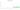SDG

Sudanese Pound

how has the value of the currency changed in the last year?USD 1 = SDG 438

How much is10 US dollars worth inSudanese pounds?
At the current exchange rate, 10 US dollars is worth 4,380 Sudanese pounds
How much is50 US dollars worth inSudanese pounds?
At the current exchange rate, 50 US dollars is worth 21,900 Sudanese pounds
How much is100 US dollars worth inSudanese pounds?
At the current exchange rate, 100 US dollars is worth 43,800 Sudanese pounds
How much is500 US dollars worth inSudanese pounds?
At the current exchange rate, 500 US dollars is worth 219,000 Sudanese pounds
How much is2,000 US dollars worth inSudanese pounds?
At the current exchange rate, 2,000 US dollars is worth 876,000 Sudanese pounds
How much is10 US dollars worth inSudanese pounds?
At the current exchange rate, 10 US dollars is worth 4,380 Sudanese pounds
How much is50 US dollars worth inSudanese pounds?
At the current exchange rate, 50 US dollars is worth 21,900 Sudanese pounds
How much is100 US dollars worth inSudanese pounds?
At the current exchange rate, 100 US dollars is worth 43,800 Sudanese pounds
How much is500 US dollars worth inSudanese pounds?
At the current exchange rate, 500 US dollars is worth 219,000 Sudanese pounds
How much is2,000 US dollars worth inSudanese pounds?
At the current exchange rate, 2,000 US dollars is worth 876,000 Sudanese pounds